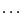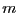Next: Parametric equations Up: Pre-processing and dealing with Previous: Pre-processing and dealing with   Contents

Pre-processing

In some cases it may be interesting to analyze a given system of equations with various initial input ranges. In the current state the system will be processed again and again for each input ranges. If this system is large this result in unnecessary computation time. When processing for the first time a system the generic analyzer will produce intermediary files in parser format in the /tmp directory. If you use the -G and -H option of the analyzer the intermediary files will be:
• Equation: the system of equation
• Hessian_Equation: the hessian equations of the system
• DimensionEq: the dimension file i.e. a file which contains a list of integer which indicates the degree of the first equation in the first unknown, in the second unknowns,, then a similar list for the second equation, and so on
• CoeffEq-: the coefficients of the equationof the system in the unknown number• Gradient_CoeffEq-: the gradient of the coefficients of the equationof the system in the unknown numberNote also that you may have to be careful when stopping the analyzer when it is generating these files. Indeed on some systems these files may be read-write protected even for the owner. If you run again the analyzer MAPLE will not be able to write the same files and will produce an error message: you will then need to remove by hand each of the created files and start again.

As soon as these files have been generated once it is not necessary to re-compute them. To indicate that these quantities have been already processed it is is sufficient to include the following sentence in the configuration file:

Directory name
Instead of computing these files the analyzer will look for them in the directory name. Thus for example after running the analyzer for the first time without this sentence in the configuration file you may analyze the same system without processing the file by indicating in your configuration file:

Directory /tmpNext: Parametric equations Up: Pre-processing and dealing with Previous: Pre-processing and dealing with   Contents
Jean-Pierre Merlet 2012-12-20